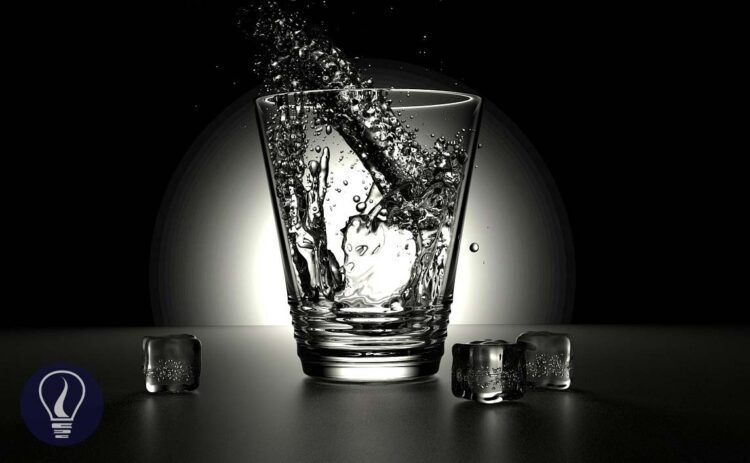More results...

Generic selectors
Exact matches only
Search in title
Search in content
Post Type Selectors

# Density and Relative DensityB.Sc (Hons, USJ) (Polymer Science and Technology, Chemistry, Physics)
Categories
Last Updated On : July 29, 2023
Published Date : July 1, 2023

## Density and Relative Density

### Density

Density is defined as the mass of a unit volume of any substance. If a substance has “m” mass and “V” volume, the density of that substance can be written as follows.

SI unit of density is kg m-3 (kilograms per cubic meter).

Density is constant for each substance. It only depends on the type of the substance. So density is an intensive property.

#### Examples of density test questions

Examples of density test questions - Question 01

At 4℃, the density of pure water is 1000 kg m-3. Express the density of water in grams per cubic meter (g cm-3)

• Solution

Examples of density test questions - Question 02

Find the mass of 500 cm3 of pure water at 4℃.

• Solution

Examples of density test questions - Question 03

The densities of two liquids of A and B are 1000 kg m-3 and 1200 kg m-3 respectively. A homogeneous mixture is obtained by mixing A and B. The final mixture has a density of 1150 kg m-3. By considering there is no volume change when mixing, find the mass and volume ratios between A and B in the mixture.

• Solution

Mass ratio

Let's take the masses of A and B are m1 and m2 respectively.

Let's apply the equation for density for the mixture.

Volume ratio

Let's take the volumes of A and B are V1 and V2 respectively.

### Relative density

The ratio between the density of any substance, to the density of water is called the “Relative density”. Relative density is a unitless and dimensionless quantity.

#### Examples of relative density test questions

Examples of relative density test questions - Question 01

Find the relative density of coconut oil. (Density of coconut oil is 890 kg m-3 and the density of water is 1000 kg m-3)

## How to calculate the density of a gas?

Also to calculate the density of a gas you can use 'The ideal gas equation'.

Where,

• P = Pressure of the gas (Pa)
• V = Volume (m3)
• n = Number of moles (mol)
• R = Universal gas constant (8.314 J mol- K-)
• T = Temperature (K)
• m = Mass of the gas (kg)
• M = Molar mass of the gas (kg mol-)
• d = Density of the gas (kg m-3)## References and Attributes

Table 01: Some data were referred from WolframAlpha.com

### Figures:

The cover image was created using an image by Zaccaria Boschetti from Pixabay